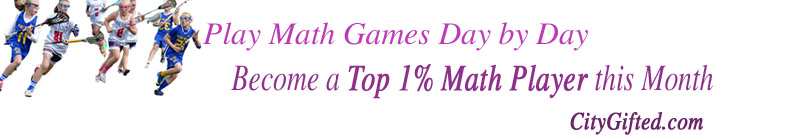SHSAT MATH PRACTICES - Goldkeyschool.com

## For Specialized High School Entrance Exam 2020

Math quiz is amusing, let's start to play this math game now

02-02-2023

Question 1

One number set is {31, 29, 9, 43, 22}, what is the average?
A) 26.8
B) 29
C) 34
D) 22.75

Question 2

The product of two positive integers is 96. Which number could be the sum of the two integers ?
A) 20
B) 19
C) 18
D) 22

Question 3

What is the greatest common factor of 54 and 702 ?

A) 27
B) 2
C) 108
D) 54

Question 4

The sum of three consecutive positive integers is 24. Which number could be the product of these three consecutive integers ?
A) 496
B) 504
C) 497
D) 511

Question 5

The product of three consecutive positive integers is 60. Which number could be the sum of the three consecutive integers ?
A) 12
B) 14
C) 11
D) 10

Question 6

One number set is {13, 109, 154, 89, 79, 56, 127, 37}, what is the median ?
A) 84
B) 83
C) 89
D) 79

Question 7

$$2^2 + 4^3 =$$
A) 16
B) 68
C) 32
D) 85

Question 8

One number set is {54, 104, 85, 82, 90, 54, 104, 54, 27, 104, 130, 46, 54, 110}, what is the mode ?
A) 103
B) 54
C) 104
D) 90

Question 9

$$2^2 + 4^3 + 2^4 =$$
A) 84
B) 101
C) 22434
D) 24

Question 10

One number set is {27, 67, 18, 27, 37, 61, 7, 27, 61, 47, 83, 40, 89, 61}, what is the range ?
A) 27
B) 37
C) 82
D) 47

Question 11

Emma played 4 spelling games and had a mean score of 150. Mike played 8 spelling games and had a mean score of 80. What was the mean of the scores for all these 12 games ?
A) 150
B) 103
C) 115
D) 80